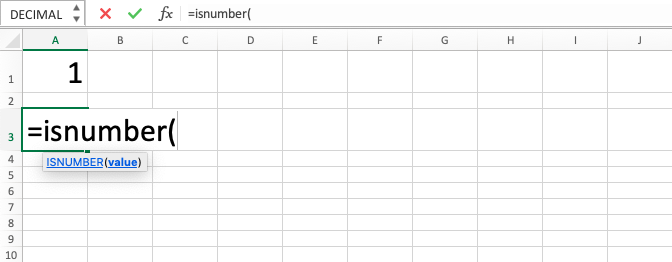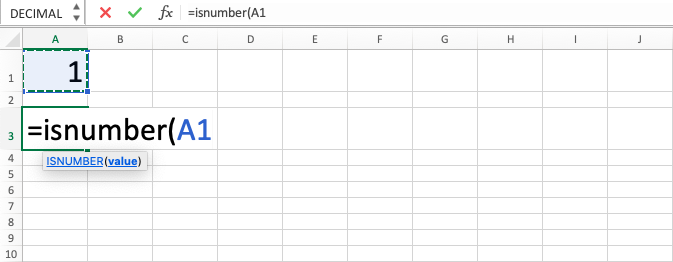ISNUMBER Excel Formula - Compute Expert

# ISNUMBER Excel Formula

In this tutorial, you will learn the ISNUMBER excel formula. ISNUMBER function in excel is useful to evaluate whether a data / a result of formula has a value with numeric data form or not. It will give the logic value of TRUE or FALSE depending on the result of the evaluation process.

## Why do We Need to Learn ISNUMBER Function in Excel?

In the data processing process on a spreadsheet, sometimes we need to check whether each data in a range has a number form or not. This is needed mainly if we want to do calculation processes in our processing. We can get errors from those calculations if the data has a form other than numbers and this, of course, must be avoided and anticipated so the processing that we do can get the right result that we need.

As an example of this in work, imagine if we want to get the average of the demand quantities history from a product in a region to be used as further analysis material to predict the demand numbers of the product in the future. The demand history data might be sourced from external sources or company databases in which the data is not inputted correctly and maybe there are some digits with the form of text on some cells in the spreadsheet. Text form digits cannot be calculated and the input of them in the calculation processes can produce errors or wrong results.

If we do not identify those data which can produce errors in the calculation processes and change them into the true digit form, then our analysis result will surely be wrong and it will give effect to the wrong prediction that we produce.

If we want to identify them but there are many cells that we need to check, then it will be very troublesome if we do it manually. To make the process faster, then you can use the ISNUMBER excel formula. ISNUMBER function in excel can give TRUE or FALSE value depending on whether the data that you check is in number form or not. It is very useful to be understood and utilized if you often deal with calculation processes in your data analysis on the spreadsheet.

## What is ISNUMBER Excel Formula?

ISNUMBER excel formula is a formula with the usage objective to check whether a data/formula result has a numeric value or not. It will give TRUE logic value if yes or FALSE if not. ISNUMBER function in excel is often used with IF formula because IF can help to produce a particular value from the TRUE or FALSE logic value.

ISNUMBER function in excel is one of the IS functions in the spreadsheet besides ISNA, ISBLANK, and the others.

Briefly, the input of this function can be explained as follows:

=ISNUMBER(value)

Note:
value = data/formula to be evaluated its result whether it has a numeric value or not

## How to Use ISNUMBER Function in Excel?

The following will explain the writing of the ISNUMBER excel formula step-by-step. ISNUMBER function in excel only needs one input which is the data/formula that you want to check.

## Using ISNUMBER Excel Formula

1. Type equal sign ( = ) in the cell where you want to put the result in2. Type ISNUMBER (can be with large and small letters) and open bracket sign after =3. Input the data or the process that produces the data / cell coordinate where it is located after open bracket sign4. Type close bracket sign5. Press Enter
6. The process is done!## Exercise

After you learn how to use ISNUMBER excel formula from above, now is the time you deepen your understanding by doing the following exercise.

### Questions

1. Is the cell content on the left numeric? Answer with TRUE or FALSE!
2. Is the cell content on the left numeric? Answer with “V” if yes or “X” if not! Use IF formula also to help you answer this question!
3. Is the cell content on the left numeric? Answer with “YES” if yes or “NO” if not! Use IF formula also to help you answer this question!

ISNUMBER function in excel will give TRUE to every data in the form of numbers including dates and currencies.

Get updated excel info from Compute Expert by registering your email. It's free!